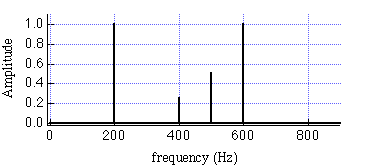TUTORIAL QUESTIONS 210-225

Back to Tutorial Listing

210.  A standing complex wave is created on a string tied at both ends by adding together several harmonics.  If the fundamental, f0, is one of the constituent harmonics making up the complex wave, then the repeat time of the standing complex wave is always equal to the period of the fundamental, T0 = 1/f0.

a) True
b) False

Fundamentals of Sound reference:Secs. 7-B, 7-D.

215.  A standing complex wave is created on a string tied at both ends by adding together several harmonics.  If the fundamental, f0, is not one of the constituent harmonics making up the complex wave, then the repeat time of the standing complex wave will not be equal to the period of the fundamental, T0 = 1/f0.

a) True
b) False

Fundamentals of Sound reference:Secs. 7-B, 7-D.

220.  Suppose you are told that the frequency spectrum of a complex sound wave is the following:The reason you can't draw a pressure versus time graph for this complex wave is:

a) We don't know the phases of the constituent frequencies
b) We don't know the constituent frequencies for the complex wave
c) We don't know the amplitudes of the constituent frequencies for the complex wave
d) The statement at the top is wrong.  You can plot the pressure versus time graph of this complex wave by knowing the frequency spectrum.

Fundamentals of Sound reference:Sec. 7-A.

225.  Suppose a piano, a clarinet, and a violin all play the same sustained note, say "middle C."  Why does the quality of the note sound different for all 3 instruments, even though the pitch of all three sounds is the same (i.e., we would hum the same note for all three sounds).

a) Because all three notes have the same repeat time, but different constituent sinusoidal frequencies making up the note.
b) Because the relative phase of the notes played by the three instruments is the same.
c) Because all three notes are made up from the same sinusoidal frequency components.
d) Because the amplitudes of all frequency components are the same for all three notes.
e) Among a)-d), two are true
f) Among a)-d), three are true
g) a)-d) are all true.

Fundamentals of Sound reference:Secs. 6-F.

Back to Tutorial Listing